# Boats and Streams - Quantitative Aptitude (MCQ) questions

Dear Readers, Welcome to Quantitative Aptitude Boats and Streams questions and answers with explanation. These Boats and Streams solved examples with shortcuts and tricks will help you learn and practice for your Placement Test and competitive exams like Bank PO, IBPS PO, SBI PO, RRB PO, RBI Assistant, LIC,SSC, MBA - MAT, XAT, CAT, NMAT, UPSC, NET etc.

After practicing these tricky Boats and Streams multiple choice questions, you will be exam ready to deal with any objective type questions.

1)   Speed of river is 6 km/hr. Speed of a motorboat in still water is 30km/hr. How much distance can it cover downstream in 24minutes?
- Published on 08 May 17

a. 9.8 km
b. 864 m
c. 12.8 km
d. 14.4 km
 Answer  Explanation ANSWER: 14.4 km Explanation: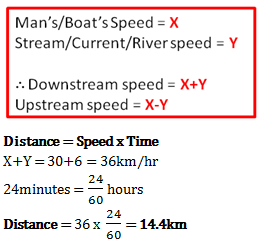2)   Find the ratio of swimming speed of Raj in still water to speed of river, if ratio of time taken to go 10km upstream to time taken to go 10km downstream is 11:5?
- Published on 08 May 17

a. 11:5
b. 4:2
c. 8:3
d. 6:5
 Answer  Explanation ANSWER: 8:3 Explanation: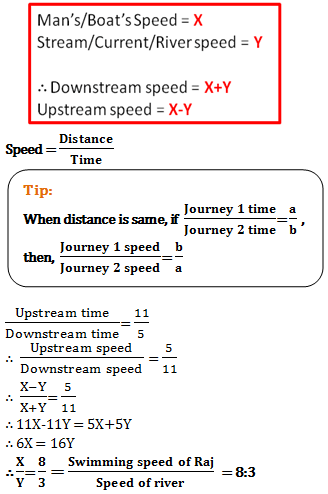3)   Guddi’s swimming speed in still water to the speed of river is 7:1. She swims 4.2 km up the river in just 14 min. How much time will Guddi take to swim 18.4 km down the river?
- Published on 03 May 17

a. 46 minutes
b. 12 minutes
c. 23 minutes
d. 11 minutes
 Answer  Explanation ANSWER: 46 minutes Explanation: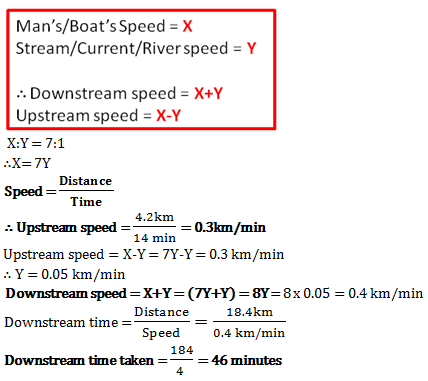4)   Michel can swim in still water at the rate of 6km per hour. After swimming in a stream, she realized that she takes twice the time to go upstream as she takes to go downstream. What is speed of the current?
- Published on 03 May 17

a. 2.25 km/hr
b. 2 km/hr
c. 4 km/hr
d. 1.5 km/hr
 Answer  Explanation ANSWER: 2 km/hr Explanation: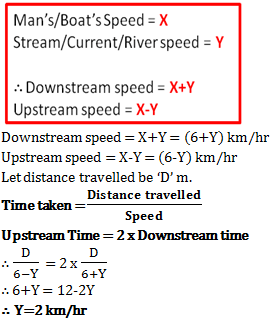5)   In 10 hours Mayur rows 30 kms upstream and 44 kms downstream. Also he travels 40 kms upstream and 55 kms downstream in 13 hours. What is speed of the boat in still water?
- Published on 13 Apr 17

a. 3 km/hr
b. 8 km/hr
c. 11 km/hr
d. 16 km/hr
 Answer  Explanation ANSWER: 8 km/hr Explanation: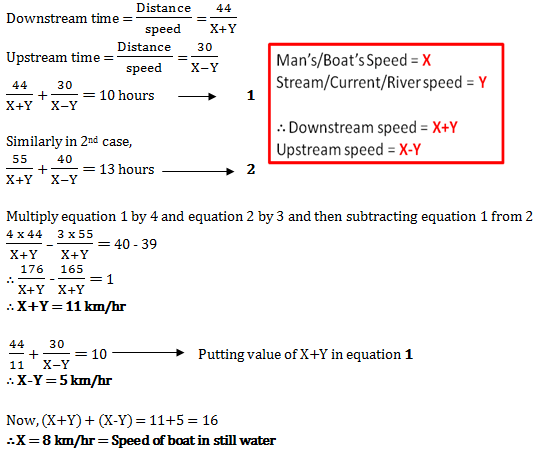6)   Raj swims 26 km downstream in same time as 14 km upstream. What is his speed in still water if speed of stream is 3 km/hr?
- Published on 12 Apr 17

a. 10 km/hr
b. 12 km/hr
c. 7 km/hr
d. 4 km/hr
 Answer  Explanation ANSWER: 10 km/hr Explanation: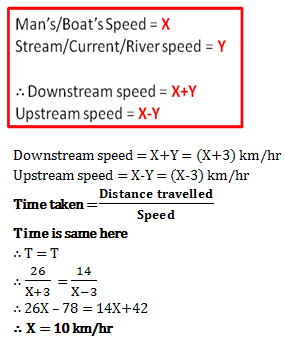7)   If upstream speed is 22 km/hr and downstream speed is 34 km/hr, what is speed of boat in still water?
- Published on 11 Apr 17

a. 28 km/hr
b. 56 km/hr
c. 17 km/hr
d. 11 km/hr
 Answer  Explanation ANSWER: 28 km/hr Explanation: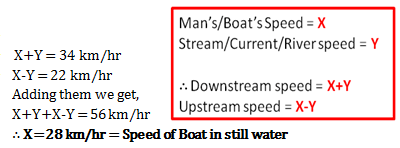8)   Consider statements A and B given below and decide whether they are enough to find the time needed for covering same distance downstream as upstream if a boat travels 48 kms upstream in 6 hours.
Statement A – Boat’s speed in still water is 10 km/hr
Statement B – Current speed is 2 km/hr

- Published on 11 Apr 17

a. Only statement B is enough
b. Any one statement if given is enough to find the answer
c. Both statements together are needed to find the answer
d. Only statement A is enough
 Answer  Explanation ANSWER: Any one statement if given is enough to find the answer Explanation: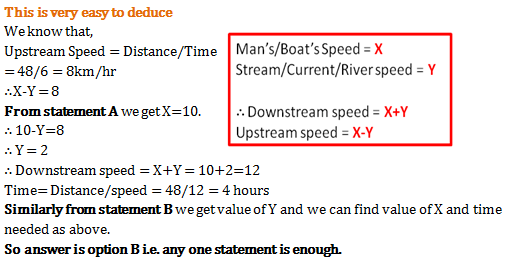9)   3 towns P, Q and R are located at the bank of a stream. Distance of Q from P and R is same. Jeet rows from P to Q and Q to P in total 10 hours. Jeet also requires 4 hours to row from P to R. If the stream flows at a consistent rate what will be the ratio of Jeet’s speed in still water and speed of stream?
- Published on 17 Mar 17

a. 6:7
b. 5:3
c. 5:2
d. 7:6
 Answer  Explanation ANSWER: 5:3 Explanation: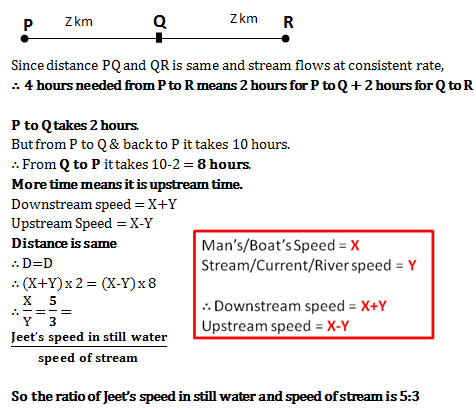10)   What will be the boat's speed in still water and speed of river, if the boat takes 12 hours to row 48 km upstream and 8 hours to row the same distance downstream?
- Published on 17 Mar 17

a. Boat = 6 km/hr; River = 4 km/hr
b. Boat = 12 km/hr; River = 6 km/hr
c. Boat = 5 km/hr; River = 1 km/hr
d. Boat = 4 km/hr; River = 3 km/hr
e. Boat = 4 km/hr; River = 3 km/hr
 Answer  Explanation ANSWER: Boat = 5 km/hr; River = 1 km/hr Explanation: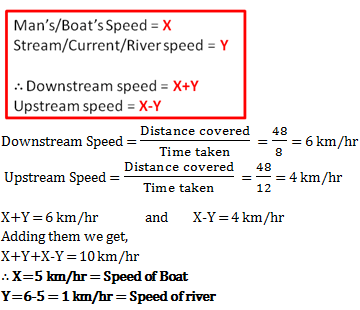1 2 3